×

Search anything:

# Hamiltonian Path

#### Algorithms hamiltonian path Graph AlgorithmsGet this book -> Problems on Array: For Interviews and Competitive Programming

Before starting with the Hamiltonian path let's get clear with what path actually is it is traversing a graph such that we do not repeat a vertex nor we repeat a edge but the starting and ending vertex must be same i.e. we can repeat starting and ending vertex only then we get a cycle.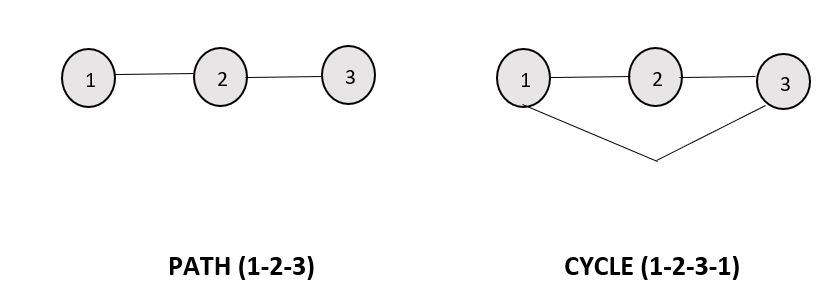### What are NP, P, NP-complete and NP-Hard problems

P : It is a set of problems that can be solved by a deterministic Turing machine in Polynomial time.

NP : It is a set of decision problems that can be solved by a Non-deterministic Turing Machine in Polynomial time. P is subset of NP (any problem that can be solved by deterministic machine in polynomial time can also be solved by non-deterministic machine in polynomial time).
It can also be said as a decision problems which can be solved by a polynomial time via a Lucky Algorithm, a magical algorithm that always makes a right guess among the given set of choices.

NP Complete : They are the hardest problems in NP set.
A decision problem L is NP-complete if:

1. L is in NP (Any given solution for NP-complete problems can be verified quickly, but there is no efficient known solution).
2. Every problem in NP is reducible to L in polynomial time (Reduction is defined below).

NP Hard : A problem is NP-Hard if it follows property 2 mentioned above, doesn’t need to follow property 1. Therefore, NP-Complete set is also a subset of NP-Hard set.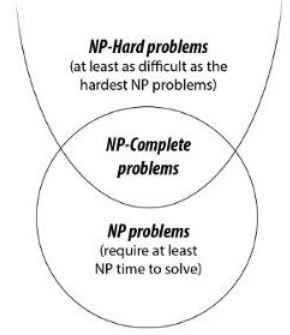Hamiltonian Path is a path in a directed or undirected graph that visits each vertex exactly once. The problem to check whether a graph (directed or undirected) contains a Hamiltonian Path is NP-complete, so is the problem of finding all the Hamiltonian Paths in a graph.

Following images explains the idea behind Hamiltonian Path more clearly :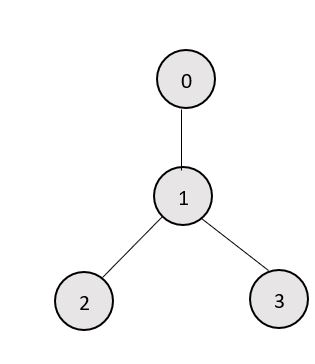Graph shown is Fig. 1 indicates does not contain any Hamiltonian Path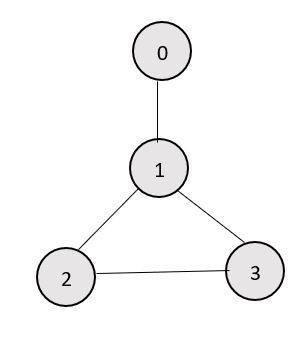Graph shown is Fig. 2 containing two Hamiltonian Paths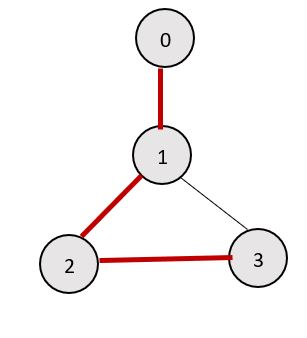==Graph shown is Fig. 3 Hamiltonian Paths which are highlighted==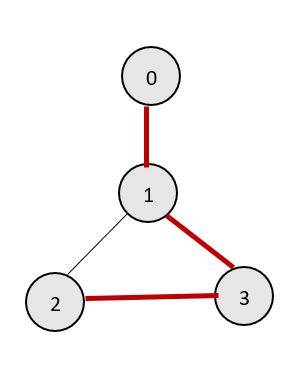Graph shown is Fig. 4 Hamiltonian Paths which are highlighted

### Some way of checking whether a graph contains a Hamiltonian Path or not

A Hamiltonian Path in a graph having N vertices is nothing but a permutation of the vertices of the graph [v1, v2, v3, ......vN-1, vN] , such that there is an edge between vi and vi+1 where 1 ≤ i ≤ N-1. So it can be checked for all permutations of the vertices whether any of them represents a Hamiltonian Path or not. For example, for the graph given in Fig. 2 there are 4 vertices, which means total 24 possible permutations, out of which only following represents a Hamiltonian Path.

``````0-1-2-3
3-2-1-0
0-1-3-2
2-3-1-0
``````

#### Following is the pseudo code of the above algorithm:

``````function check_all_permutations(adj[][], n)
for i = 0 to n
p[i]=i
while next permutation is possible
valid = true
for i = 0 to n-1
valid = false
break
if valid == true
return true
p = get_next_permutation(p)
return false
``````

The function get_next_permutation(p) generates the lexicographically next greater permutation than p.

Following is the C++ implementation:

``````bool check_all_permutations(bool adj[][MAXN], int n){
vector<int>v;
for(int i=0; i<n; i++)
v.push_back(i);
do{
bool valid=true;
for(int i=0; i<v.size()-1; i++){
valid=false;
break;
}

}
if(valid)
return true;
}while(next_permutation(v.begin(), v.end()));
return false;
}
``````

### Time Complexity

Time complexity of the above method can be easily derived.
For a graph having N vertices it visits all the permutations of the vertices, i.e. N! iterations and in each of those iterations it traverses the permutation to see if adjacent vertices are connected or not i.e N iterations, so the complexity is O( N * N! ).

### Code to print the Hamiltonian Path

``````#include <iostream>
#include <vector>
using namespace std;

// data structure to store graph edges
struct Edge {
int src, dest;
};

// class to represent a graph object
class Graph
{
public:
// An array of vectors to represent adjacency list

// Constructor
Graph(vector<Edge> const &edges, int N)
{
// resize the vector to N elements of type vector<int>

// add edges to the undirected graph
for (unsigned i = 0; i < edges.size(); i++)
{
int src = edges[i].src;
int dest = edges[i].dest;

}
}
};

void printAllHamiltonianPaths(Graph const& g, int v, vector<bool>
visited, vector<int> &path, int N)
{
// if all the vertices are visited, then
// Hamiltonian path exists
if (path.size() == N)
{
// print Hamiltonian path
for (int i : path)
cout << i << " ";
cout << endl;

return;
}

// Check if every edge starting from vertex v leads
// to a solution or not
{
// process only unvisited vertices as Hamiltonian
// path visits each vertex exactly once
if (!visited[w])
{
visited[w] = true;
path.push_back(w);

// to solution or not
printAllHamiltonianPaths(g, w, visited, path, N);

// Backtrack
visited[w] = false;
path.pop_back();
}
}
}

// main function
int main()
{
// consider complete graph having 4 vertices
vector<Edge> edges = {
{0, 1}, {0, 2}, {0, 3}, {1, 2}, {1, 3}, {2, 3}
};

// starting node
int start = 0;

// Number of vertices in the graph
int N = 4;

// create a graph from edges
Graph g(edges, N);

// add starting node to the path
vector<int> path;
path.push_back(start);

// mark start node as visited
vector<bool> visited(N);
visited[start] = true;

printAllHamiltonianPaths(g, start, visited, path, N);

return 0;
}
``````

Output :

``````0 1 2 3
0 1 3 2
0 2 1 3
0 2 3 1
0 3 2 1
0 3 1 2
``````

### Question

#### Which of the following algorithm can be used to solve the Hamiltonian path problem efficiently?

branch and bound
iterative improvement
divide and conquer
greedy algorithm
The Hamiltonian path problem can be solved efficiently using branch and bound approach. It can also be solved using a backtracking approach.#### Harshita Sahai

Maintainer at OpenGenus | Previously Software Developer, Intern at OpenGenus (June to August 2019) | B.Tech in Information Technology from Guru Gobind Singh Indraprastha University (2017 to 2021)